Courses
Courses for Kids
Free study material
Offline Centres
More

# NCERT Solutions for Class 6 Maths Chapter 13 - ExerciseLast updated date: 04th Dec 2023
Total views: 529.5k
Views today: 14.29k

## NCERT Solutions for Class 6 Maths Chapter 13 Symmetry (Ex 13.1) Exercise 13.1

Free PDF download of NCERT Solutions for Class 6 Maths Chapter 13 Exercise 13.1 (Ex 13.1) and all chapter exercises at one place prepared by an expert teacher as per NCERT (CBSE) books guidelines. Class 6 Maths Chapter 13 Symmetry Exercise 13.1 Questions with Solutions to help you to revise complete Syllabus and Score More marks. Register and get all exercise solutions in your emails.

Every NCERT Solution is provided to make the study simple and interesting on Vedantu. Subjects like Science, Maths, English,Hindi will become easy to study if you have access to NCERT Solution for Class 6 Science , Maths solutions and solutions of other subjects.

## Access NCERT Solutions for Maths Class 6 Chapter 13 - Symmetry

Exercise 13.1

1. List any four symmetrical from your home or school.

Ans: These objects can be flipped horizontally and end up in the same result.

a) Shirt

b) A door

c) Carpets

d) Tv

2. For the given figure, which one is the mirror line, \${l_1}\$ or \${l_2}\$?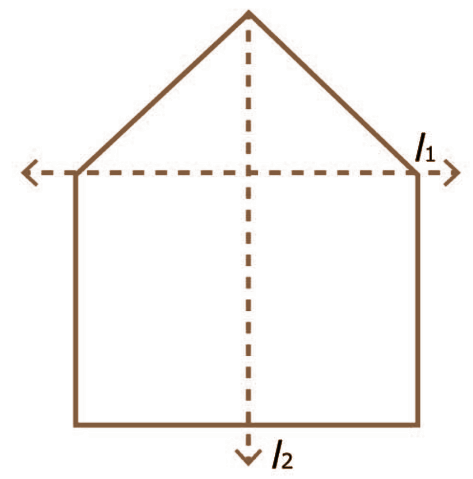Ans: \${l_2}\$ is the mirror line because \${l_2}\$ divides the figure symmetrically.

3. Identify the shapes given below. Check whether they are symmetric or not. Draw the line of symmetry as well.

a)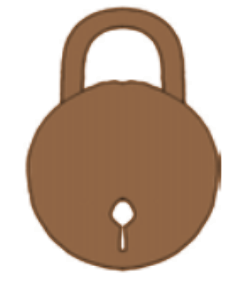Ans: The given shape is symmetric and the line of symmetry is drawn.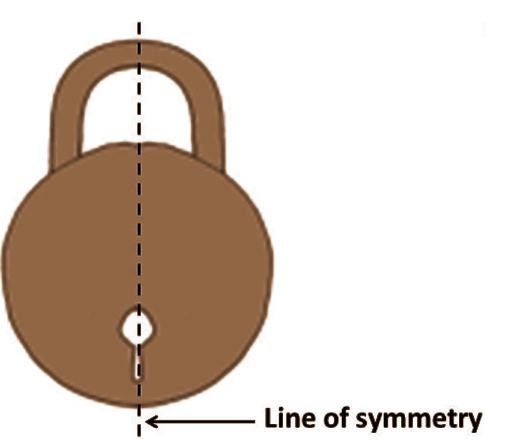b)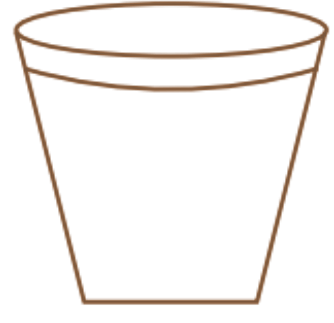Ans: The given shape is symmetric and the line of symmetry is drawn.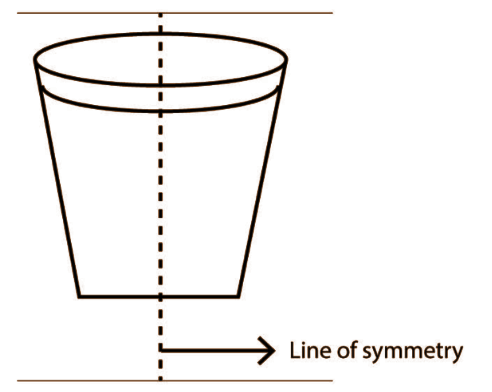c)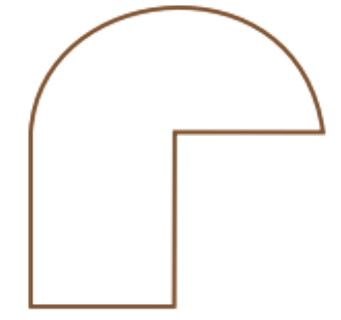Ans: No, it is not symmetrical hence there is no line of symmetry in it.

d)Ans:   The given shape is symmetric and the line of symmetry is drawn.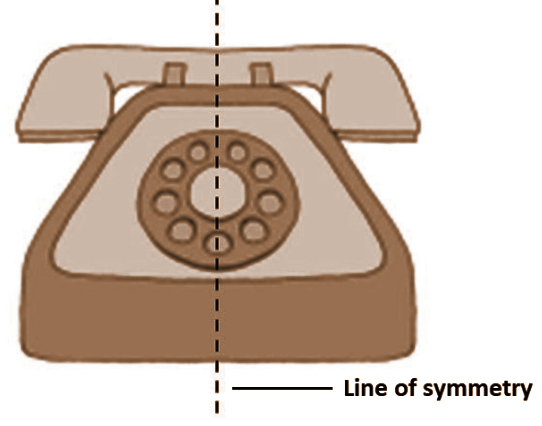e)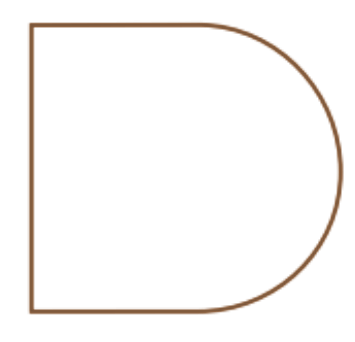Ans: The given shape is symmetric and the line of symmetry is drawn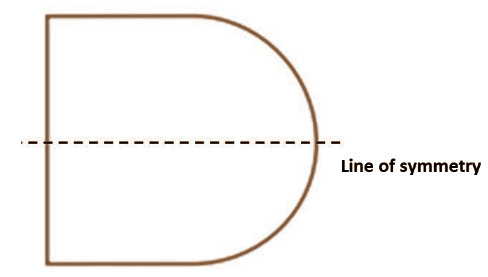f)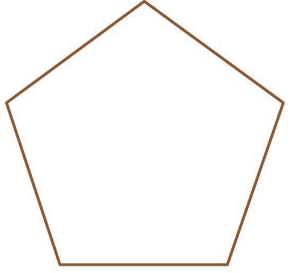Ans: The given shape is symmetric and the line of symmetry is drawn.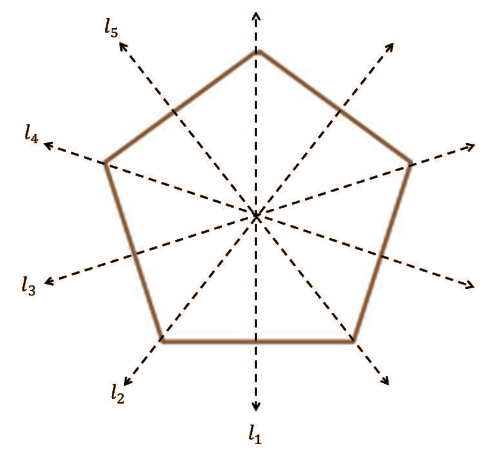4. Copy the following on a square paper. A square paper is what you would have used in your arithmetic notebook in earlier classes. Then complete them such that the dotted line is the line of symmetry.

a)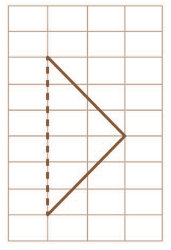Ans: The shape is drawn such that the dotted line is the line of symmetry of the shape.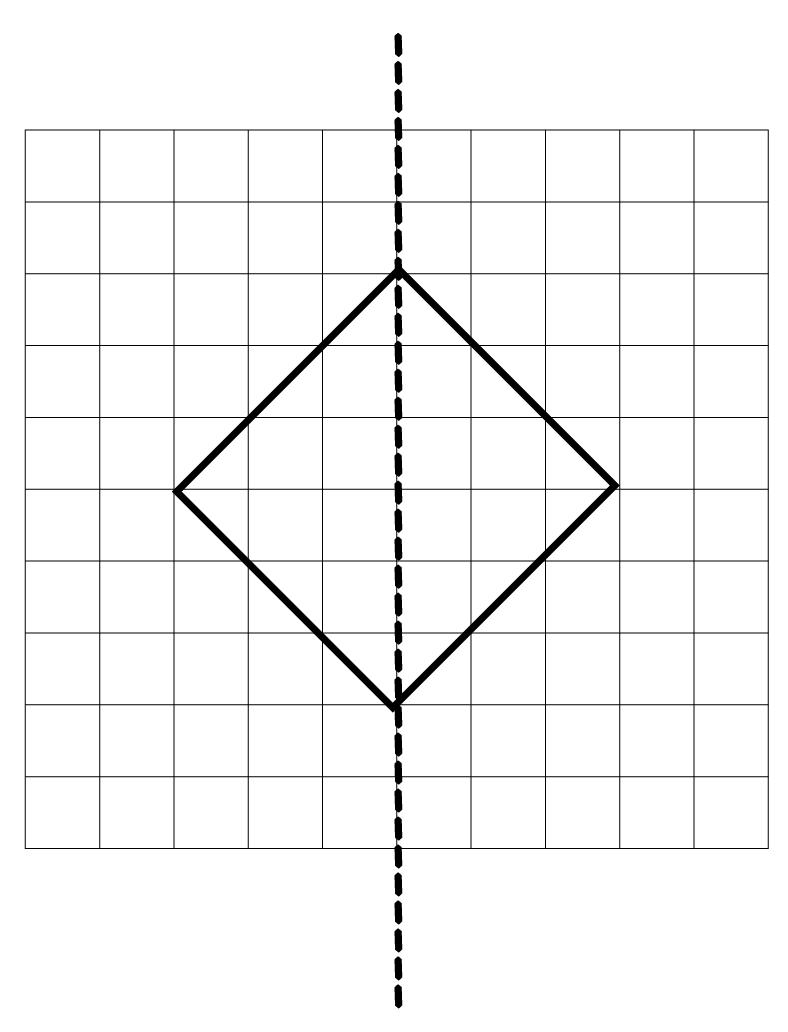b)

Ans: The shape is drawn such that the dotted line is the line of symmetry of the shape.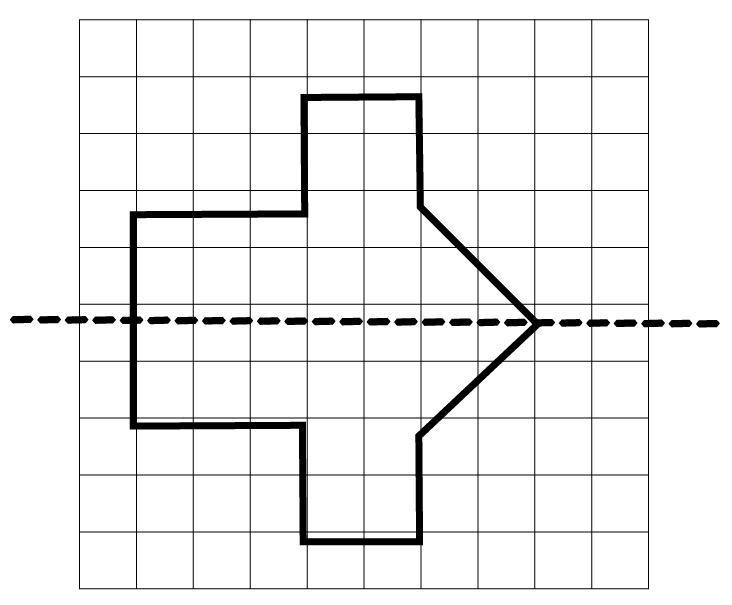c)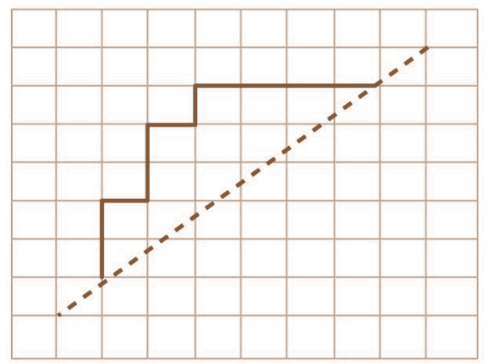Ans: The shape is drawn such that the dotted line is the line of symmetry of the shape.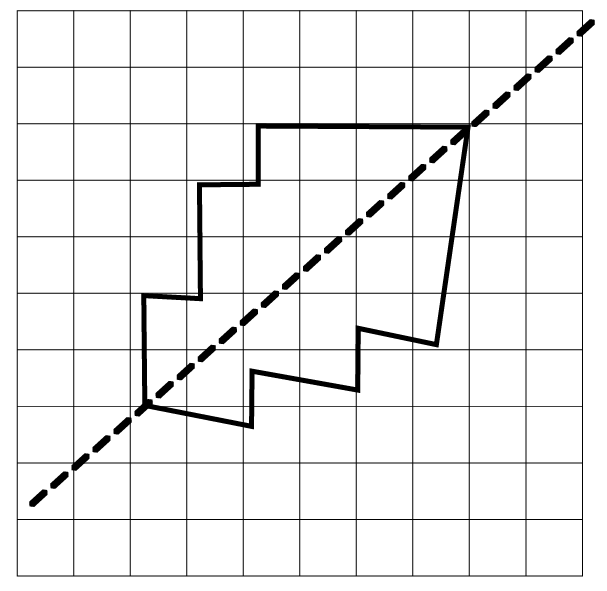d)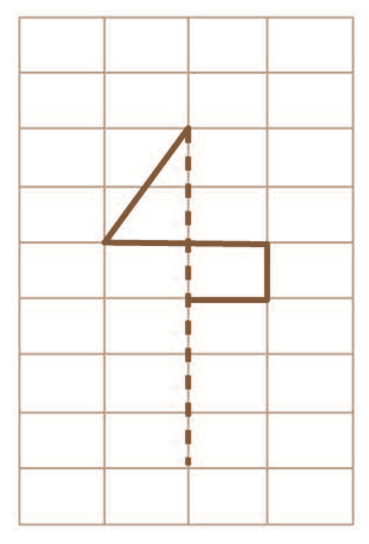Ans: The shape is drawn such that the dotted line is the line of symmetry of the shape.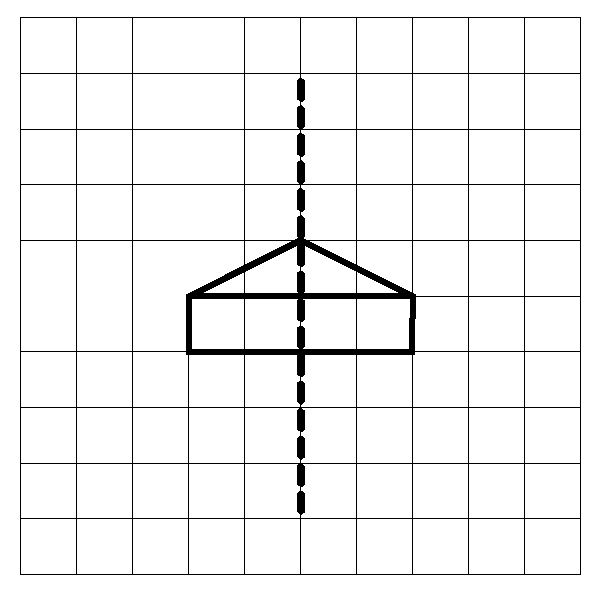e)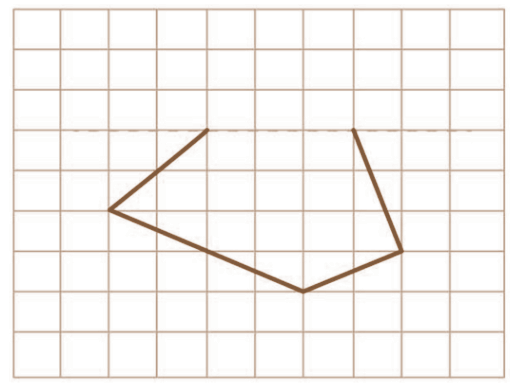Ans: The shape is drawn such that the dotted line is the line of symmetry of the shape.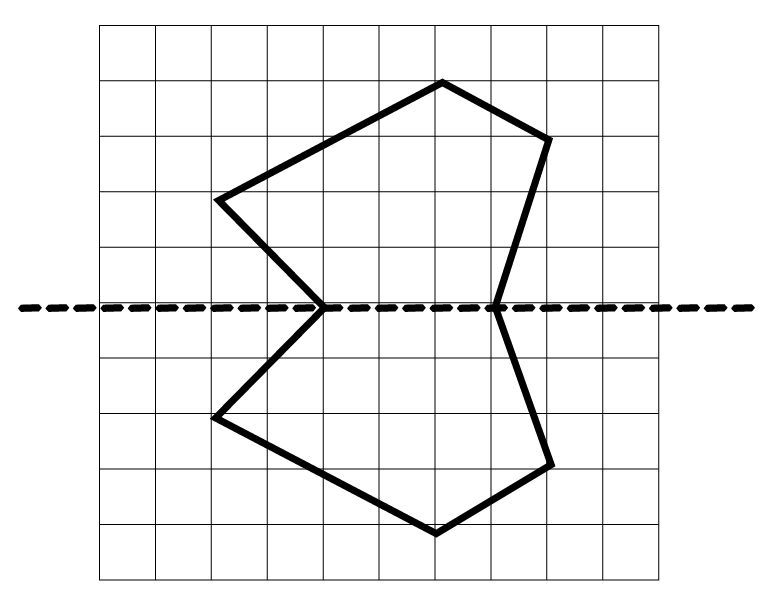f)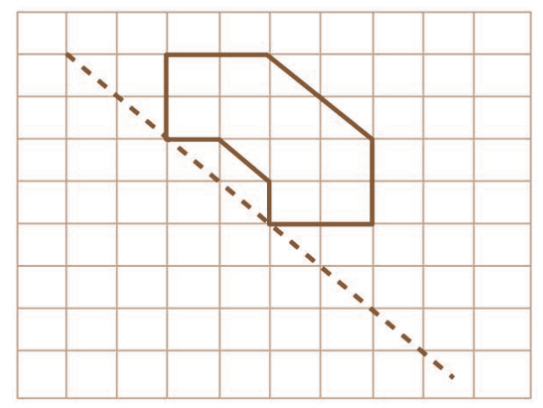Ans: The shape is drawn such that the dotted line is the line of symmetry of the shape.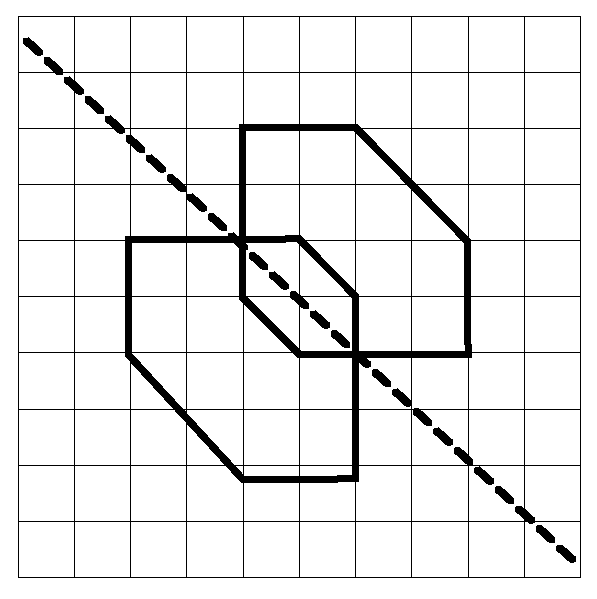5. In the figure, \$l\$ is the line of symmetry. Complete the diagram to make it symmetric.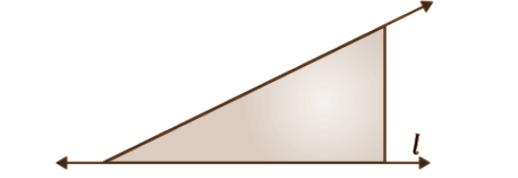Ans: To make the diagram symmetric, draw the line similar to that of the given figure.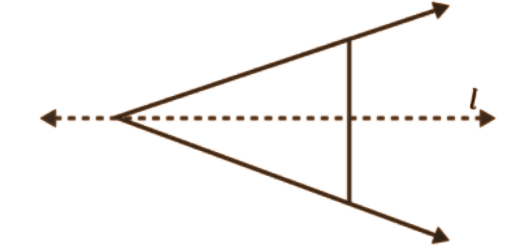Now the figure is symmetric.

6. In the figure, \$l\$ is the line of symmetry. Draw the image of the triangle and complete the diagram, so that it becomes symmetric.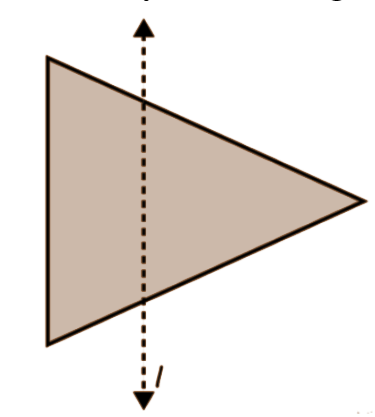Ans: To draw the symmetric of diagram just draw the inverse of the given triangle with respect to the line \$l\$.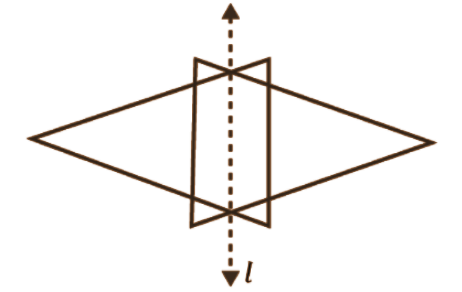## NCERT Solutions for Class 6 Maths Chapter 13 Symmetry Exercise 13.1

Opting for the NCERT solutions for Ex 13.1 Class 6 Maths is considered as the best option for the CBSE students when it comes to exam preparation. This chapter consists of many exercises. Out of which we have provided the Exercise 13.1 Class 6 Maths NCERT solutions on this page in PDF format. You can download this solution as per your convenience or you can study it directly from our website/ app online.

Vedantu in-house subject matter experts have solved the problems/ questions from the exercise with the utmost care and by following all the guidelines by CBSE. Class 6 students who are thorough with all the concepts from the Maths textbook and quite well-versed with all the problems from the exercises given in it, then any student can easily score the highest possible marks in the final exam. With the help of this Class 6 Maths Chapter 13 Exercise 13.1 solutions, students can easily understand the pattern of questions that can be asked in the exam from this chapter and also learn the marks weightage of the chapter. So that they can prepare themselves accordingly for the final exam.

Besides these NCERT solutions for Class 6 Maths Chapter 13 Exercise 13.1, there are plenty of exercises in this chapter which contain innumerable questions as well. All these questions are solved/answered by our in-house subject experts as mentioned earlier. Hence all of these are bound to be of superior quality and anyone can refer to these during the time of exam preparation. In order to score the best possible marks in the class, it is really important to understand all the concepts of the textbooks and solve the problems from the exercises given next to it.

Do not delay any more. Download the NCERT solutions for Class 6 Maths Chapter 13 Exercise 13.1 from Vedantu website now for better exam preparation. If you have the Vedantu app in your phone, you can download the same through the app as well. The best part of these solutions is these can be accessed both online and offline as well.

## FAQs on NCERT Solutions for Class 6 Maths Chapter 13 - Exercise

Q1. How many questions are present in NCERT Solutions for Class 6 Maths Chapter 13 Symmetry?

Ans: Class 6 Maths Chapter 13 has three exercises in total -

• Ex 13.1 - 6 questions based on the basic introduction to symmetry.

• Ex 13.2 - 8 questions based on different types of lines of symmetry, i.e, one line of symmetry, two lines of symmetry, three lines of symmetry, and no lines of symmetry.

• Ex 13.3 - 3 questions containing the further explanation of symmetry with various figures.

Q2. What do you understand by ‘Line of Symmetry’?

Ans: When a figure is folded in such a way that the figure is divided into two equal halves, the line that splits the figure into two halves is called the line of symmetry. The line of symmetry has various types:

• 1 line of symmetry - For example, in an isosceles triangle.

• 2 lines of symmetry - For example, in a rectangle.

• 3 lines of symmetry - For example, in an equilateral triangle.

Q3. Is it possible to find 'Symmetry' concepts in everyday life?

Ans: The presence of symmetry can be seen in a variety of places, from the tiniest to the largest of objects :

• The left and right sides of most butterflies' wings are identical.

• The reflection of trees and mountains in clean water.

• Taj Mahal is built symmetrically.

• Honeycombs are symmetrical.

• Human faces are mostly symmetrical.

• Pizzas are cut into symmetries.

The list of symmetry examples is truly endless.

Q4. How to download NCERT Solutions for Class 6 Maths Chapter 13?

Ans: You can download the NCERT Solutions of every exercise of Class 6 Maths Chapter 13 from the website of Vedantu. To have more easy access, you can download the Vedantu app on your device. These NCERT Solutions are provided in downloadable PDF format so that you can access them offline too. These PDFs are available for free of cost. These Solutions are prepared by the expert team of Vedantu for your effective preparations.

Q5. Why should I choose Vedantu to study Maths?

Ans: Mathematics appears to be a subject that requires more effort and practice. You can only excel in Maths if you grasp all the fundamental concepts. You will undoubtedly require an additional study guide along with the textbooks, to assist you in learning the concepts more effectively. Vedantu addresses these concerns and provides you with unique learning resources. To avail these resources,visit Vedantu today.